## Abstract

An experimental investigation on the thermal efficiency and thermal resistance with respect to the inclined angle using heat pipe was carried out. The working fluids used for this are Al2O3 nanofluid and deionized water. The performance of heat pipe in terms of overall heat transfer coefficient and thermal resistance is quantified by varying the volume of working fluid and the performance parameters are contemplated. For this purpose Al2O3 nanoparticles with a density of 9.8 g/cm3 and a volume concentration of 1% are used as the working fluid in experimental heat pipe. The performance of heat pipe was evaluated by conducting experiments with different thermal loads and different angle of inclinations. Thermocouples are used to record the temperature distribution across the experiment. Volume of nanoparticles in the base fluid and the consequence of filling ratio on the thermal resistance of the nanofluids are investigated. The results provide evidence that the suspension of Al2O3 nanoparticles in the base fluid increases the thermal efficiency of heat pipe and can be used in practical heat exchange applications.

## Keywords

Heat pipe; Angle of inclination; Thermal resistance; Thermal efficiency

## Nomenclature

A- area, m2

I- C, A

K- thermal conductivity, W/m K

Q- heat supplied to the heat pipe, W

R- thermal resistance, K/W

Te- temperature of evaporator region, °C

Tc- condenser surface temperature, °C

ν- kinematic diffusivity

μ- viscosity of the base liquid

ρf- liquid density

bf- base fluid

c- condenser

e- evaporator

HP- heat pipe

nf- nanofluid

np- nanoparticle

## 1. Introduction

Studies revealed the fact that thermal energy is precious among all the energies in earth. The transfer of heat in thermal management systems results in increase in cost and decrease in the overall efficiencies. The technology with high capacity of cooling in less time with more efficient procedures called transfer of heat through heat pipes was implemented nowadays. This technology was given priority than heat conducted by metals such as silver and copper because of its noiseless operations and weightless fixed components. This technology is foremost important in the design of various engineering components such as radiator, shell and tube heat exchanger to enhance the heat transfer coefficient.

Heat transfer is one of the most important industrial processes. In any industrial facilities heat must be efficiently managed by adding, removing or moving in the relevant sectors. Conventional heat transfer fluids such as water, ethylene glycol (EG), and pumping oil, have not shown sufficient capability for cooling applications due to their poor thermal performance. It has been already demonstrated that adding solid particle to these traditional fluids could enhance their thermal performance. However, still these suspensions with micrometer or larger size particles are not efficient choice for the high-tech applications such as microelectronics, data centers and micro-channels. Therefore, development of highly efficient heat transfer fluids for solving the drawback of conventional fluids has become one of the most important priorities in the cooling industries. In the last decade, nanoscience and nanotechnology have offered new solution by introducing nanofluids which may assist to enhance heat transfer fluids’ performance.

It’s an apparatus with a sealed cylindrical pipe having a wick like structure inside it through which heat transfer fluids such as ethylene glycol, water, and engine oils are used commonly. In terms of comparison of thermal conductivity, it is obvious that a metallic solid will be higher than that of a fluid. Experiments on years prove that combination of particles of nanometer size with the heat transferring fluid will increase the overall thermal conductivity. In recent years combinations such as water with alumina and water with copper oxide have been tested in heat pipes and the results are analyzed by researchers because of their higher value of k.

Leong et al.  focused on the application of ethylene glycol based copper nanofluids in an automotive cooling system and obtained relevant input data, nanofluid properties and empirical correlations from the literature to investigate the heat transfer enhancement of an automotive car radiator operated with nanofluid-based coolants. They observed that the coolants’ pressure drop increased with the addition of copper nanoparticles and heat transfer rate is increased with increase in volume concentration of nanoparticles. Ali et al.  reported for water based ZnO nanofluids to enhance the heat transfer performance of a car radiator in different volumetric concentrations (0.01%, 0.08%, 0.2% and 0.3%) and observed significant increase in heat transfer rates using ZnO water nanofluids compared to base fluid. The best heat transfer enhancement up to 46% is achieved using 0.2% volume of nanofluid.

Chougule and Sahu et al.  studied experimentally the convective heat transfer enhancement of carbon nanotube (CNT)-water nanofluid inside an automobile radiator and reported as for 1.0 vol.% nanoparticle concentration and coolant flow rate of 5 l/min, the maximum enhancement in heat transfer of FCNT–water nanofluid was found to be 90.76% higher compared with water. Hussein et al.  have investigated the friction factor and forced convection heat transfer enhancements of SiO2 nanoparticle dispersed in water as a base fluid conducted in a car radiator experimentally and numerically for four different concentrations of nanofluids in the range of 1–2.5 vol% and found that the maximum values of friction factor increased to 22% for SiO2 nanoparticles dispersed in water with 2.5% volume concentration. Naraki et al.  investigated experimentally the overall heat transfer coefficient of CuO/water nanofluids is under laminar flow regime and the results are statistically analyzed using Taguchi method by implementing Qualitek-4 software. The maximum value of the overall heat transfer coefficient with nanofluid, effect of each operating parameter on the overall heat transfer coefficient and the optimum values of each parameter are determined.

Delavari and Hashemabadi  have presented numerical study on simulated turbulent and laminar flow heat transfer in nanofluids (Al2O3 particles in water and ethylene glycol-based fluid) passing through a flat tube in 3D using computational fluid dynamics (CFD) for single and two-phase approaches. The numerical results were the same as for the experimental data, indicating that increasing the concentration of nanoparticles in the base fluid increased the heat transfer coefficient and the Nusselt number. Elias et al.  present new findings on the thermal conductivity, viscosity, density, and specific heat of Al2O3 nanoparticles dispersed into water and ethylene glycol based coolant used in car radiator. They suggested that it would be better if the effect of particle size and shape could be considered with the variation of temperature and particle concentrations for the investigation of the thermo-physical properties.

Mousa  has thermally tested the heat pipe with two different working fluids: pure water and Al2O3–water based on the nanofluid by comparing the available data with the experimental data. Kumaresan and Venkatachalapathy reviewed the influence of various factors such as heat pipe tilt angle, charged amount of working fluid, nanoparticles type, size, and mass/volume fraction and its effect on the improvement of thermal efficiency, heat transfer capacity and reduction in thermal resistance. Annamalai and Ramalingam  had conducted experiments to determine the surface and vapor temperature at steady and transient conditions for two different input powers in the evaporator section and cooling the condenser section by air by means of computational fluid dynamics analysis.

Senthilkumar et al.  analyzed the thermal performances of the cylindrical heat pipe with wire mesh are experimentally investigated using the DI water, copper nanofluid and copper nanofluid with aqueous solution of n-hexanol as working fluids in terms of their thermal performance, the thermal efficiency and the thermal resistances. Do et al.  conducted experiment on the screen mesh wicked heat pipes using Al2O3 nanofluids and showed that the heat pipe performance has been increased up to 40%. Wong and Ho  conducted experiments on horizontal heat pipes to investigate the effects of Al2O3 or Cu nanoparticles laden in mesh-wick evaporator. Senthilkumar et al.  perform a comparative study of heat pipe performance using the aqueous solution of n-Pentanol with water at various inclinations and presented the results to demonstrate the merits and suitability of the aqueous solution of n-Pentanol as a working fluid for heat pipe.

Vassallo et al.  compared the heat transfer performance of silica nano-solutions and silica micro-solutions under atmospheric pressure with a 0.4 mm NiCr wire submerged in each solution with pure water.

## 2. Experimental setup and procedure

### 2.1. Nanofluids and its preparations

Typically solid particles ranging from 1 to 100 nm made-up of composite materials in solid–liquid condition called nanofluids are used by suspended with working fluid in heat pipes. Reducing the ratio of mass and volume of the solids and liquids causes the nanofluid to occur in a free stable state for a long time. The viscosity of the fluid was increased resulting in unchanged chemical reactions in the base fluid. Due to its increased thermal properties, nanofluids are used in various types of heat exchangers as thermo-fluids. Precious heat energy and the components used to transfer heat are conserved by these fluids by increasing heat transfer coefficient. The characteristics of heat transfer such as specific heat (c) density (ρ), thermal conductivity (K), viscosity (μ) influencing the heat transfer depend on the operating temperature.

Both the values of K and μ increase by using nanofluids compared to base fluid. The effective value of K for nanofluids can be determined by the equation

 $k_{eff}={\frac {k_{p}+2k_{bf}+2\varphi (k_{p}-k_{bf})}{k_{p}+2k_{bf}-2\varphi (k_{p}-k_{bf})}}k_{bf}$
(1)

An average size of 50 nm Al2O3 nanoparticles with a density of 9.8 g/cm3 was obtained from OttoKemi Chemicals Private Ltd. which is a Mumbai based company is utilized to determine the characteristics of heat transfer. It is then dispersed in water by using ultrasonic vibrator to obtain 1% volume concentration for 6 h in order to achieve uniform dispersion and stable suspension.

For Al2O3 nanoparticles with 1% volume fraction the ratio of Knf/Kbf was found to be 1.039 ± 0.003 which will be increased to 1.121 ± 0.004 if the volume fraction is 3%. This shows the variation ranges from 1.003 ± 0.008 to 1.204 ± 0.010 for the ratio of Knf/Kbf proving that the increase in volume fraction will increase the thermal conductivity. Fig. 1 shows the SEM image of Al2O3 nanoparticle for the size of 50 nm.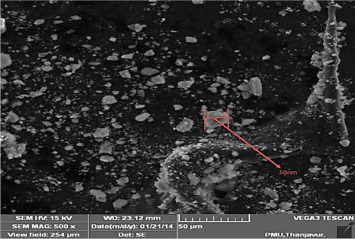Figure 1. SEM image for Al2O3 nanoparticle of 50 nm.

Continuous collisions between the np and the molecules of bf cause the Brownian motion which is the random motion of np within the bf. Increase in the temperature intensifies the Brownian motion, as per the kinetic theory of particles. The particles suspended in the bf are kept unsettled due to gravity by means of Brownian motion. Nano convection also increases the transport of energy in nanofluid system. Heat transfer rate due to convection can be calculated by convection diffusivity which is the kinematic diffusivity (v) of the liquid.

 $\nu =\mu /{\rho }_{f}$

### 2.2. Experimental setup

It consists of a thick walled pipe of copper having a length of 1.1 m with 0.19 m inner diameter and 0.21 m outer diameter. Figure 2 and Figure 3 shows the ultrasonic bath and photograph of the experimental setup. Fig. 4 shows the schematic representation of the heat pipe showing that the first 0.35 m and the last 0.35 m are the evaporator and condenser sections. Between these sections the pipe is thermally insulated for a length of 0.4 m called adiabatic section. The setup consists of 0.000183 m diameter of screen mesh made of stainless steel. The whole pipe is filled with the analyzing fluid of 40 ml corresponding to the required fluid to be filled in the evaporator section. Six constant thermocouples having uncertainty of ±0.1 °C are used to determine the HP’s temperature distribution by placing at a fixed distance.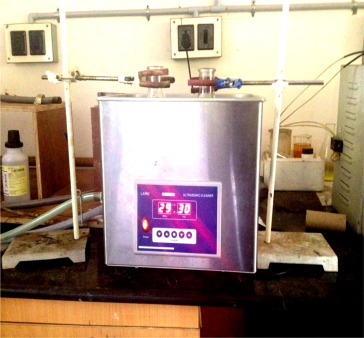Figure 2. Ultrasonic bath.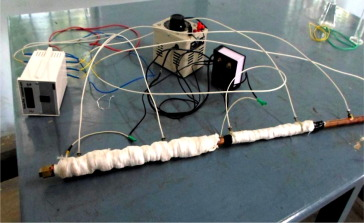Figure 3. Photograph of the experimental setup.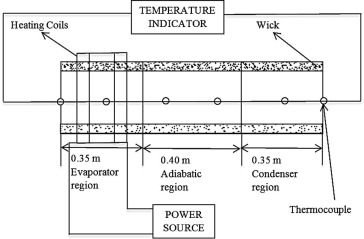Figure 4. Schematic of the experimental setup.

Neglecting the loss of heat in the evaporator and condenser sections, the readings are measured by applying electrical power. Electrical heater coils with a supply of 230 V AC are used along the evaporator section to increase the temperature of the working fluid. As the whole arrangement obeys the laws of thermodynamics the heat pipe will transfer the heat supplied by the coils to the copper pipe and the working fluid. This results in increase in temperature of the copper pipe which must be condensed by the condenser to avoid critical situations. Two thermocouples equally placed are used to measure the evaporator and condenser’s average temperatures.

### 2.3. Experimental procedure

The whole arrangement of thermocouple and heat pipe is positioned and the data loggers are connected to the corresponding thermocouples. Initially the dissolved gases inside the heat pump are evacuated using a vacuum pump. By filling of 10 ml (25% fill ratio) of the working fluid, the evaporator section is supplied by heat through the heater coils and the temperature for steady state condition was monitored. This procedure is repeated for different volumes of working fluids in the evaporator region of heat pipe in the ratios of 50%, 75% and 100%. The heat pipe efficiency increases with increasing tilt angle because the gravitational force has a significant effect on the flowing of working fluid between evaporator section and condenser section. Hence the readings are measured for different angle of inclinations 0°, 30°, 60°, and 90° and analyzed. The whole procedure has been done for DI water and Al2O3 nanofluids and the readings are tabulated for analysis.

## 3. Results and discussion

### 3.1. Effect of angle of inclination and fill ratio on the thermal efficiency of the heat pipe

The variations in volume of working fluid in the evaporator section with respect to the inclined angles will cause a change in thermal efficiency and this is schematically represented for Al2O3 nanofluids in Figure 5 and Figure 6. The output ratio of the cooling capacity of the working fluid along the condenser region to the input heat supplied along the evaporator region results in the thermal efficiency. Mani et al. (2012) studied the addition of copper oxide nanoparticles to the base fluid results in the maximum thermal efficiency at 75% volume of working fluid filled in the evaporator region and proved about 14.3% increase in thermal efficiency compared to DI water at an angle of 30°.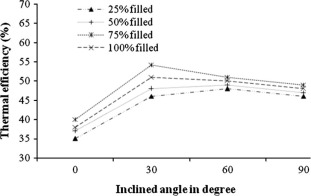Figure 5. Inclined angle vs thermal efficiency for DI water.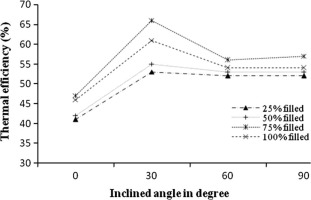Figure 6. Inclined angle vs thermal efficiency for Al2O3 nanofluids.

The fluid absorption capacity that depends on the volume of the working fluid causes the raise in thermal efficiency of the heat pipes. Higher volume of the working fluid reduces the vapor space in the condenser region which further causes the reduction in rate of heat transfer in the evaporator regions working fluid. This results in an increase in thermal efficiency to a value of 66% by filling 75% of working fluid which is 11.2% greater than that of DI water. Compared to copper oxide nanofluid the increase in thermal efficiency by using Al2O3 nanofluids is 2.5% less and hence thermophoresis can be done for future enhancement of heat transfer by Al2O3 nanofluids. The efficiency is high at 30° of inclination and it is gradually reduced after that because of the orientation. Depending on conditions, a heat pipe can be operated in horizontal position or in vertical position. For the horizontal position of a heat pipe, gravity has no effect. But in vertical position gravity can assist or oppose to the operation of the heat pipe. The tilt of a heat pipe is classified into two types: favorable tilt and adverse tilt. Favorable tilt is the tilt position where gravity assists heat pipe operation. In favorable tilt, condenser is positioned above evaporator. By this way, liquid return from condenser to evaporator is assisted by gravity. Therefore, capillary pumping pressure can overcome more pressure losses and this increases the heat transfer capacity of the heat pipe, in terms of capillary limit. Other type is adverse tilt. In this tilt condition, evaporator is positioned above condenser. Therefore, the liquid in the condenser shall overcome gravity force to return to evaporator. This creates extra drag for capillary pumping pressure to overcome. As a result, heat transfer capacity of the heat pipe decreases. Therefore, it is preferable for a heat pipe to operate in favorable tilt position.

### 3.2. Effect of angle of inclination and fill ratio on the thermal resistance of the heat pipe

Continuous operations of heat exchangers will increase the resistance to heat transfer in heat exchangers. Normally used heat transfer fluids such as water, air, oil or gases in heat exchanger which contains less value of K have limits to the heat transfer rate. This can be reduced by enhancing techniques by either active or passive methods.

The value for R can be found by the ratio between the temperature difference between the evaporator and condenser regions to the net heat supplied to the heat pipes evaporator section.

 $R=T_{e}-T_{c}/Q$

The variations found by these values for DI water and Al2O3 nanofluids at different inclined angles are shown in Figure 7, Figure 8, Figure 9 and Figure 10.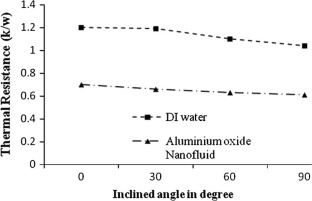Figure 7. Inclined angle vs thermal resistance for different inclined angles at 25%.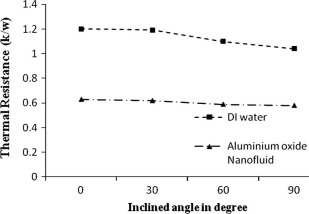Figure 8. Inclined angle vs thermal resistance for different inclined angles at 50%.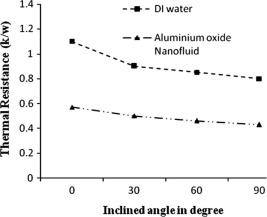Figure 9. Inclined angle vs thermal resistance for different inclined angles at 75%.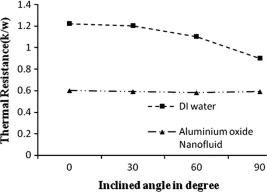Figure 10. Inclined angle vs thermal resistance for different inclined angles at 100%.

Figure 7 and Figure 8 represent the values of thermal resistance in terms of inclined angle of degree for the fill ratios of 25% and 50%. From the graphs it is visible that for both the nf and DI water the thermal resistance for 25% fill ratio is slightly less than that of 50% fill ratio.

Figure 9 and Figure 10 represent the values of thermal resistance in terms of inclined angle of degree for the fill ratios of 75% and 1000%. From the graphs it is visible that by an increase in inclined angle, it is clearly visible from the graphs that there will be decrease in value of R of the heat pipe. The value of R will be less in heat pipes which use Al2O3 nanofluids as working fluid compared to DI water. From Fig. 7 the value of thermal resistance is 0.45 K/W for the filled ratio of 75% Al2O3 nanofluid and the maximum decrease of about 52% thermal resistance was obtained compared to DI water. The value of k has considerable increase by using nanoparticles in the base fluid. Mani et al. (2012) analyzed copper oxide nanofluid and found the value of thermal resistance is 0.33 K/W for the filled ratio of 75% which is 0.12 K/W less than that of the value of R by using Al2O3 nanofluid. Also the maximum decrease of 62% was found in copper oxide nanofluid which is 10% more than Al2O3 nanofluid.

## 4. Conclusion

In this paper, we have experimentally investigated the effective thermal efficiency and thermal resistance of water-based nanofluids containing Al2O3 nanoparticles (Al2O3/water nanofluids). For this purpose, we synthesized Al2O3 nanoparticles having an average diameter of 50 nm and with volume concentration of 1% using a two-step method with ultrasonication. For parameters such as angle of inclination and fill ratio the experiment was conducted and the results are summarized as follows:

• For the fill ratio of 75% and for the inclined angle of 30° both the DI water and the Al2O3 nanofluid show a descent increase in thermal efficiency.
• The value of thermal efficiency by using Al2O3 nanofluid in the heat pipe is higher than the value obtained by using DI water.
• Though the thermal resistance value of 0.45 K/W with a maximum decrease of 62% is slightly higher than that of the values obtained by using copper oxide nanofluid, still Al2O3 nanofluid can be used for heat exchanging equipments by typical augmentation process.
• It is clearly visible that the suspension of nanoparticles in the base fluid causes a tremendous improvement in heat transfer rate. Further, the design of heat pipe in COMSOL multiphysics can be done and the results can be numerically analyzed with these experimental results in future.

### Document informationPublished on 12/04/17

Licence: Other

### Document Score0

Views 22
Recommendations 0